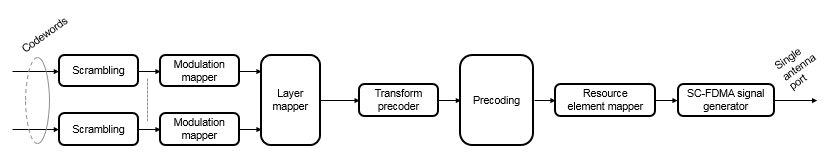ltePSSCHDecode

PSSCH decoding

Description

example

[softbits,symbols] = ltePSSCHDecode(ue,sym) returns a vector of log-likelihood ratio (LLR) soft bits and the intermediate QPSK or 16QAM modulation symbols for the specified UE settings structure and modulated PSSCH symbols.

The PSSCH decoder performs the inverse of the ltePSSCH function processing, as defined in TS 36.211 , Section 9.3. The ltePSSCHDecode processing includes SC-FDMA transform deprecoding, symbol demodulation, and PSSCH-specific descrambling. For more information, see Physical Sidelink Shared Channel Decoding.

Examples

collapse all

Demodulate PSSCH symbols plus noise for an SL-SCH codeword created by encoding a vector of information bits. Plot the noisy RE symbols, the symbols prior to QPSK demodulation, and the resulting LLR soft bits.

Create a UE settings structure

Specify normal cyclic prefix and 16-QAM modulation.

ue = struct('CyclicPrefixSL','Normal');
ue.RV = 0;
ue.Modulation = '16QAM';
ue.NSAID = 255;
ue.NSubframePSSCH = 0;

Generate symbols to recover

• Specify the codeword length to use for the SL-SCH. Choose a length that is a multiple of 12 symbols for normal cyclic prefix and has 4 bits per symbol for 16-QAM modulation. Pick a standard number of resource blocks, such as 10.

• Create the SL-SCH codeword.

• Create the PSSCH symbols and add noise.

codewordlength = 5760; % (12 symbols)(4 bps)(12 REperRB)(10 PRB)
cw = lteSLSCH(ue,codewordlength,ones(100,1));

sym = ltePSSCH(ue,cw);
rxsym = sym + 0.1*randn(size(sym));

Recover the soft bits representing the transmitted SL-SCH codeword. Compare the soft bits to the transmitted codeword.

[rxcw,rxmodsym] = ltePSSCHDecode(ue,rxsym);
isequal(cw,rxcw>0)
ans = logical
0

Using a random noise seed and the level of noise added sometimes results in decoding errors. If the comparison returns '1' there were no decoding errors. If the comparison returns '0' there were decoding errors.

Plot the received and recovered signals.

subplot(2,2,1)
plot(rxsym,'o')
title('PSSCH Encoded Symbols + Noise')

subplot(2,2,2)
plot(rxmodsym,'o')
title('Decoded Symbols')

subplot(2,2,[3,4])
plot(rxcw)
title('Decoded Soft Bits')Input Arguments

collapse all

User equipment settings, specified as a parameter structure containing these fields:

Sidelink mode, specified as 'D2D' or 'V2X'.

Data Types: char | string

Cyclic prefix length, specified as 'Normal' or 'Extended'.

Data Types: char | string

Modulation type, specified as 'QPSK' or '16QAM'.

Data Types: char | string

Sidelink group destination identity, specified as an integer in the interval [0, 255].

This field is the lower eight bits of the full 24-bit ProSe Layer-2 group destination ID. This field and the NSubframePSSCH field control the value of the scrambling sequence at the start of each subframe. This field is required only for D2D sidelink.

Data Types: double

V2X scrambling identity, specified as an integer scalar. NXID is the 16 bit CRC associated with the PSCCH SCI grant. It is only required for V2X sidelink.

Data Types: double

PSSCH subframe number in the PSSCH subframe pool, specified as an integer scalar. (${n}_{\text{ssf}}^{\text{PSSCH}}$)

NSubframePSSCH and NSAID control the values of the scrambling sequence. It is only required for D2D sidelink.

Data Types: double

Data Types: struct

Encoded modulated PSSCH symbols, specified as an NRE-by-1 column vector. NRE is the number of RE in a subframe associated with the PSSCH allocation for normal and extended cyclic prefix (including the SC-FDMA guard symbol) and NPRB resource blocks.

NRE is NPRB × 144 for D2D normal cyclic prefix or NPRB × 120 for D2D extended cyclic prefix and V2X. NPRB is the number of physical resource blocks (PRB) used for transmission.

The function requires the contents of all PSSCH resource elements to be input, including those in the last guard symbol. For more information, see Physical Sidelink Shared Channel Decoding.

Data Types: double
Complex Number Support: Yes

Output Arguments

collapse all

Log-likelihood ratio (LLR) soft bits, returned as a vector with Nbps × NRE softbits. Nbps is the number of bits per symbol. PSSCH modulation is either QPSK (2 bits per symbol) or 16 QAM (4 bits per symbol). NRE is the number of PSSCH resource elements in the subframe. The LLR of the punctured soft bits associated with the last SC-FDMA symbol are set to 0.

Decoded modulated PSSCH symbols, returned as a column vector with NRE elements. NRE is the number of PSSCH resource elements in the subframe. For more information, see Physical Sidelink Shared Channel Decoding.

collapse all

The physical sidelink shared channel (PSSCH) decoder performs the inverse of the ltePSSCH function processing. For more information, see Physical Sidelink Shared Channel Processing. PSSCH decoding includes SC-FDMA transform deprecoding, symbol demodulation, and PSSCH-specific descrambling.Physical sidelink shared channel (PSSCH) processing includes PSSCH-specific scrambling, QPSK or 16-QAM modulation, and SC-FDMA transform precoding. PSSCH processing follows the processing steps used for PUSCH, with variations defined in TS 36.211, Section 9.3.For PSSCH, the input codeword length is Mbits = NRE × Nbps, where Nbps is the number of bits per symbol. PSSCH modulation is either QPSK (2 bits per symbol) or 16 QAM (4 bits per symbol).

The number of PSSCH resource elements (NRE) in a subframe is NRE = NPRB × NREperPRB × NSYM and includes symbols associated with the sidelink SC-FDMA guard symbol.

• NPRB is the number of physical resource blocks (PRB) used for transmission.

• NREperPRB is the number of resource elements in a PRB. Each PRB has 12 resource elements.

• NSYM is the number of SC-FDMA symbols in a PSSCH subframe, including symbols associated with the sidelink SC-FDMA guard symbol. The number of SC-FDMA symbols in a PSSCH subframe is 12 for D2D normal cyclic prefix or 10 for D2D extended cyclic prefix and V2X.

The info structure output by ltePSSCHIndices provides Mbits and NRE as info.G and info.Gd respectively.

The scrambling sequence generator is initialized with ${c}_{\text{init}}={n}_{\text{ID}}^{\text{X}}×{2}^{14}+{n}_{\text{ssf}}^{\text{PSSCH}}×{2}^{9}+510$ at the start of every PSSCH subframe. For D2D sidelink, ${n}_{\text{ID}}^{\text{SA}}$ is the destination identity (NSAID) obtained from the sidelink shared channel. For V2X, ${n}_{\text{ID}}^{\text{SA}}$ is the V2X scrambling identity (NXID). ${n}_{\text{ssf}}^{\text{PSSCH}}$ is the subframe number in the PSSCH subframe pool (NSubframePSSCH).

ltePSSCH requires CyclicPrefixSL to deduce the number of resource blocks allocated for SC-FDMA precoding symbols.

 3GPP TS 36.211. “Evolved Universal Terrestrial Radio Access (E-UTRA); Physical Channels and Modulation.” 3rd Generation Partnership Project; Technical Specification Group Radio Access Network. URL: https://www.3gpp.org.